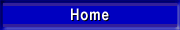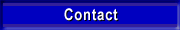ON-LINE MANUAL
Definitions

## 10. Definitions and terms

Acceleration
The rate of change of velocity with time (Dv/Dt) usually along a specific axis, expressed in "g".

Amplitude
The magnitude of variation from a base value (normally zero). It is used for displacement, velocity or acceleration. Excursion is double the Amplitude (Peak to peak). The value is useful in the balancing procedure to reduce the vibration forces generated by rotation by adjusting a mass in the rotating element.

Critical Frequency
The particular resonant ferquency at which a damage (or degrading in performance) to the installation occurs.

Cycle
The complete sequence of instantaneous values of a periodic event that occur during one period.

Damping
The dissipation of vibratory energy with motion or time. Critical damping is the value of damping that provides most rapid response to a step function without overshot.

Forced Vibration
The vibration motion of a system is said to be forced if it is caused by some mechanical excitation.

Free Vibration
Occurs without forcing, as after a string is plucked.

Frequency
The reciprocal value of the period. The bestknown unit is Hertz (Hz), which represents cycles per second. When referreing to vibrators, cycles per minute or revolutions per minute (r.p.m.) are common.

Impact
Impact is a single collision between masses at least one of which is in motion.

Impulse
The intergral force within a time window.

Intensity
The severity of a vibration or shock. Similar to amplitude but without specific connotation.

Isolation
Isolation is the reduction in severity of motion, usually attained by proper use of resilient support.

Jerk
Refers to the ratio of change of acceleration with time (Da/Dt).

Natural Frequency
The frequency of free vibration of a system.

Oscillation
The variation with time of a quantity, such as force, pressure, displacement or velocity. Usually some regularity such as sinusoidal or complex vinration is implied.

Peak
The extreme value of a varying quantity measured from the base or mean value.

Period
The period of a periodic vibration is the smallest interval of time in which the vibration repeats itself.

Response
The vibration motion, force or energy of a mechanical system that results from some mechanical input to that system.

Resonance
In a true Single-Degree-of-Freedom system resonance exists when any change of excitation frequency causes a decrease in its response. Therefore, resonance represents the maximum response of the sprung mass, if frequency is varied while input force is kept constant.

Transient Vibration
The temporarily sustained vibration of a mechanical system.

Vibrometer
A device to measure the frequency of a vibrating machine. Usually an extendable spring that can be adjusted to come in resonance at defined lengths.